2021-08-06
2021-08-06

## Introduction

Eigenvectors and eigenvalues have many important applications in computer vision and machine learning in general. Well-known examples are PCA (Principal Component Analysis) for dimensionality reduction or EigenFaces for face recognition. An interesting use case of eigenvectors and eigenvalues is also illustrated in my post about error ellipses. Furthermore, eigendecomposition forms the base of the geometric interpretation of covariance matrices, discussed in a more recent post. In this article, I will provide a gentle introduction to this mathematical concept, and will show how to manually obtain the eigendecomposition of a 2D square matrix.

An eigenvector is a vector whose direction remains unchanged when a linear transformation is applied to it. Consider the image below in which three vectors are shown. The green square is only drawn to illustrate the linear transformation that is applied to each of these three vectors.

Eigenvectors (red) do not change direction when a linear transformation (e.g. scaling) is applied to them. Other vectors (yellow) do.

The transformation, in this case, is a simple scaling with factor 2 in the horizontal direction and factor 0.5 in the vertical direction, such that the transformation matrixis defined as: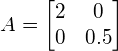.

A vector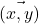is then scaled by applying this transformation as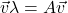. The above figure shows that the direction of some vectors (shown in red) is not affected by this linear transformation. These vectors are called eigenvectors of the transformation, and uniquely define the square matrix. This unique, deterministic relation is exactly the reason that those vectors are called ‘eigenvectors’ (Eigen means ‘specific’ in German).

In general, the eigenvectorof a matrixis the vector for which the following holds:

(1)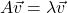where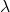is a scalar value called the ‘eigenvalue’. This means that the linear transformationon vectoris completely defined by.

We can rewrite equation (1) as follows:

(2)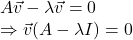where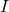is the identity matrix of the same dimensions as.

However, assuming thatis not the null-vector, equation (2) can only be defined ifis not invertible. If a square matrix is not invertible, that means that its determinant must equal zero. Therefore, to find the eigenvectors of, we simply have to solve the following equation:

(3)In the following sections, we will determine the eigenvectors and eigenvalues of a matrix, by solving equation (3). Matrixin this example is defined by:

(4)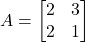## Calculating the eigenvalues

To determine the eigenvalues for this example, we substitutein equation (3) with equation (4) and obtain:

(5)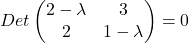Calculating the determinant gives:

(6)To solve this quadratic equation in, we find the discriminant:Since the discriminant is strictly positive, this means that two different values forexist:

(7)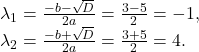We have now determined the two eigenvaluesand. Note that a square matrix of size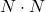always has exactly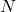eigenvalues, each with a corresponding eigenvector. The eigenvalue specifies the size of the eigenvector.

## Calculating the first eigenvector

We can now determine the eigenvectors by plugging the eigenvalues from equation (7) into equation (1) that originally defined the problem. The eigenvectors are then found by solving this system of equations.

We first do this for eigenvalue, in order to find the corresponding first eigenvector: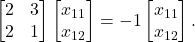Since this is simply the matrix notation for a system of equations, we can write it in its equivalent form:

(8)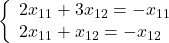and solve the first equation as a function of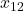, resulting in:

(9)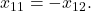Since an eigenvector simply represents an orientation (the corresponding eigenvalue represents the magnitude), all scalar multiples of the eigenvector are vectors that are parallel to this eigenvector and are therefore equivalent (If we would normalize the vectors, they would all be equal). Thus, instead of further solving the above system of equations, we can freely choose a real value for eitheror, and determine the other one by using equation (9).

For this example, we arbitrarily choose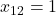, such that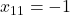. Therefore, the eigenvector that corresponds to eigenvalueis

(10)## Calculating the second eigenvector

Calculations for the second eigenvector are similar to those needed for the first eigenvector;
We now substitute eigenvalueinto equation (1), yielding:

(11)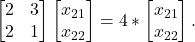Written as a system of equations, this is equivalent to:

(12)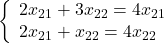Solving the first equation as a function ofresults in:

(13)We then arbitrarily choose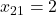and find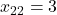. Therefore, the eigenvector that corresponds to eigenvalueis

(14)A large set of data can be represented as a matrix and we need to somehow compress the columns of the sparse matrix to speed up our calculations. Plus if we multiply a matrix by a vector then we achieve a new vector. The multiplication of a matrix by a vector is known as transformation matrices. We can transform and change matrices into new vectors by multiplying a matrix with a vector. The multiplication of the matrix by a vector computes a new vector. This is the transformed vector. Hold that thought for now!

The new vector can be considered to be in two forms:

1. Sometimes, the new transformed vector is just a scaled form of the original vector. This means that the new vector can be re-calculated by simply multiplying a scalar (number) by the original vector; just as in the example of vectors A and B above.
2. And other times, the transformed vector has no direct scalar relationship with the original vector which we used to multiply to the matrix.

If the new transformed vector is just a scaled form of the original vector then the original vector is known to be an eigenvector of the original matrix. Vectors that have this characteristic are special vectors and they are known as eigenvectors. Eigenvectors can be used to represent a large dimensional matrix.

Therefore, if our input is a large sparse matrix M then we can find a vector o that can replace the matrix M. The criteria is that the product of matrix M and vector o should be the product of vector o and a scalar n: M * o = n* o

This means that a matrix M and a vector o can be replaced by a scalar n and a vector o.

In this instance, o is the eigenvector and n is the eigenvalue and our target is to find o and n.

Therefore an eigenvector is a vector that does not change when a transformation is applied to it, except that it becomes a scaled version of the original vector.

Eigenvectors can help us calculate an approximation of a large matrix as a smaller vector. There are many other uses which I will explain later on in the article.

Eigenvectors are used to make linear transformation understandable. Think of eigenvectors as stretching/compressing an X-Y line chart without changing their direction.

Eigenvalue— The scalar that is used to transform (stretch) an Eigenvector.

Eigenvalues and eigenvectors are all about constructing one vector with one value to represent a large matrix. If a square matrix has a size n then we will get n eigenvalues and as a result, n eigenvectors will be computed to represent the matrix.

# Visual Explanation:

#### Linear Transformations

Suppose A is a matrix of size m×n. Given a vector

Then T is a linear transformation from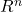toHow is this used? Suppose you want to scale a 2d vector by a factor of 2 along the x-axis and 3 along the y-axis. Say the vector v is [1, 4] then after scaling it should be [2, 12]. This can be done as follows:

This may look trivial for one vector. But suppose if you have n number of 2d vectors that you want to scale, you can transform all of them at once by only one matrix multiplication operation. Linear Transformations are widely used in the field of Computer Graphics, Game Engines, Statistics, etc.

This operation is not only limited to scaling, but we can use linear transformation matrices for flipping vectors, rotating vectors, shearing vectors, etc.

### Going back to Eigenvectors and Eigenvalues

Suppose we have a square represented in 2d space where every point on the square is a vector of which I will be using only 3 vectors as shown below.

Suppose we scale the square by a factor of 2 along the y-axis as shown below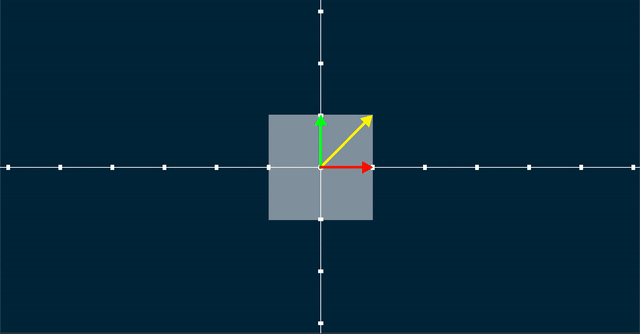Scaling by a factor of 2 along the y-axis

If you notice the red vector has the same scale and direction after the linear transformation. The green vector changes in scale but still has the same direction. Whereas the yellow vector neither has the same scale but also its angle with the x-axis increased, hence its direction also changed. If we look closely, apart from the red vector and the green vector all the other vectors’ directions changed. Hence we can say the red and green vectors are special and they are characteristic of this linear transform. These vectors are called eigenvectors of this linear transformation. And their change in scale due to the transformation is called their eigenvalue. For the red vector the eigenvalue is 1 since its scale is constant after and before the transformation, whereas for the green vector, its eigenvalue is 2 since it is scaled up by a factor of 2.

Let’s have a look at another linear transformation where we shear the square along the x-axis.

If you guessed the red vector to be the eigenvector, you’re right and its eigenvalue is 1.

What if we rotate the square 90 degrees clockwise?

Here there are no eigenvectors (Academic people will argue that there are complex eigenvectors in this case, but they are far away from the scope of this article so let’s stick with this case having no eigenvectors for simplicity). What if instead of 90 degrees we rotated the square by 180 degrees?

Here all the vectors along with the three colored vectors are eigenvectors with an eigenvalue of -1.

Let’s have a look at a special case where we scale the square equally along the x and y-axis.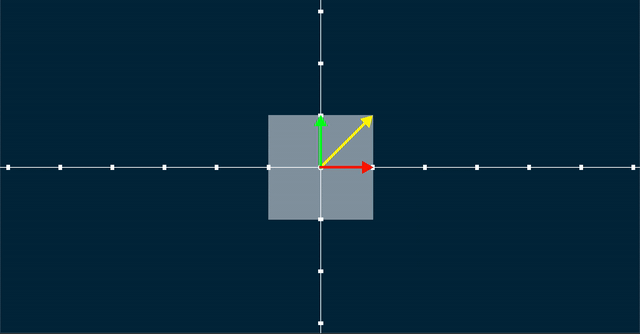Scaling equally along the x and y-axis

Here all the vectors are eigenvectors and their eigenvalue would be the scale factor.

Now let’s go back to Wikipedia’s definition of eigenvectors and eigenvalues:

If T is a linear transformation from a vector space V over a field F into itself and v is a vector in V that is not the zero vector, then v is an eigenvector of T if T(v) is a scalar multiple of v. This condition can be written as the equation

T ( v ) = λ v

where λ is a scalar in the field F, known as the eigenvaluecharacteristic value, or characteristic root associated with the eigenvector v.

Let’s see how the equation works for the first case we saw where we scaled a square by a factor of 2 along the y axis where the red vector and green vector were the eigenvectors.Scaling by a factor of 2 along the y-axis

Before the linear transformation:

After the linear transformation:

We can show that after the linear transformation using the equation:

Let’s scale the problem up to 3 dimensions. Suppose we rotate a cube along the z-axis then the vector along the z-axis would be the eigenvector with an eigenvalue of 1.

It gets hard to visualize and figure out the eigenvectors if we go above 3 dimensions. Even in some cases in 2d or 3d, it’s not that easy. So how do we figure out the eigenvectors?

We know that for any eigenvector v,

Let’s say the transformation matrix is A. Therefore,

From both of the above equations, we can deduce that,

Moving the terms together,

Now λ is just a scalar. We can take v as common out if we can convert it into a matrix since A is a matrix. To do so we can multiply λ with an identity matrix I. Therefore,

Now for the right-hand side to be 0 either (A-λI) should be 0 or/and v should be 0. But if you remember from the definition an eigenvector is a non-zero vector. So (A-λI) should always be 0 for v to be an eigenvector. We can calculate whether a matrix operation is 0 by calculating its determinant.

Therefore,

Let’s see if this works using the same example of scaling a square by a factor of 2 along the y-axis.

Here the transformation matrix A can be shown as:

Now we know that,

Putting in the values of A and solving further:

We know that,

Solving for λ = 1 we get,

This means for any vector where v2=0 that vector is an eigenvector with eigenvalue 1. It’s true for any vertical vector, which in our case was the red vector.

Solving for λ = 2 we get:

This means for any vector where v1=0 that vector is an eigenvector with eigenvalue 2. It’s true for any vertical vector, which in our case was the green vector.

Applications:

1. There are multiple uses of eigenvalues and eigenvectors: Eigenvalues and Eigenvectors have their importance in linear differential equations where you want to find a rate of change or when you want to maintain relationships between two variables. Think of eigenvalues and eigenvectors as providing a summary of a large matrix.

2. We can represent a large set of information in a matrix. Performing computations on a large matrix is a very slow process. To elaborate, one of the key methodologies to improve efficiency in computationally intensive tasks is to reduce the dimensions after ensuring most of the key information is maintained. Hence, one eigenvalue and eigenvector are used to capture key information that is stored in a large matrix. This technique can also be used to improve the performance of data churning components.

3. Component analysis is one of the key strategies that is utilized to reduce dimension space without losing valuable information. The core of component analysis (PCA) is built on the concept of eigenvalues and eigenvectors. The concept revolves around computing eigenvectors and eigenvalues of the covariance matrix of the features.

4. Additionally, eigenvectors and eigenvalues are used in facial recognition techniques such as EigenFaces.

5. They are used to reduce dimension space. The technique of Eigenvectors and Eigenvalues is used to compress the data. As mentioned above, many algorithms such as PCA rely on eigenvalues and eigenvectors to reduce the dimensions.

6. Eigenvalues are also used in regularisation and they can be used to prevent overfitting.

Eigenvectors and eigenvalues are used to reduce noise in data. They can help us improve efficiency in computationally intensive tasks. They also eliminate features that have a strong correlation between them and also help in reducing over-fitting.

6. Occasionally we gather data that contains a large amount of noise. Finding important or meaningful patterns within the data can be extremely difficult. Eigenvectors and eigenvalues can be used to construct spectral clustering. They are also used in singular value decomposition.

7. We can also use eigenvectors to rank items in a dataset. They are heavily used in search engines and calculus.

8. Lastly, in non-linear motion dynamics, eigenvalues and eigenvectors can be used to help us understand the data better as they can be used to transform and represent data into manageable sets.

Having said that, it can be slow to compute eigenvectors and eigenvalues. The computation is O(n³)

### Calculate Eigenvalues and Eigenvectors in Python

Although we don’t have to calculate the Eigenvalues and Eigenvectors by hand, it is important to understand the inner workings to be able to confidently use the algorithms. Furthermore, It is very straightforward to calculate eigenvalues and eigenvectors in Python.

We can use numpy.linalg.eig module. It takes in a square matrix as the input and returns eigenvalues and eigenvectors. It also raises an LinAlgError if the eigenvalue computation does not converge.

import numpy as np
from numpy import linalg as LA
input = np.array([[2,-1],[4,3]])
w, v = LA.eig(input)
print(w)
print(v)


## Conclusion

In this article, we reviewed the theoretical concepts of eigenvectors and eigenvalues. These concepts are of great importance in many techniques used in computer vision and machine learning, such as dimensionality reduction using PCA, or face recognition using EigenFaces.

Reference:

https://www.visiondummy.com/

https://medium.com/fintechexplained/what-are-eigenvalues-and-eigenvectors-a-must-know-concept-for-machine-learning-80d0fd330e47

https://towardsdatascience.com/eigenvectors-and-eigenvalues-all-you-need-to-know-df92780c591f##### Amir Masoud Sefidian
Machine Learning Engineer